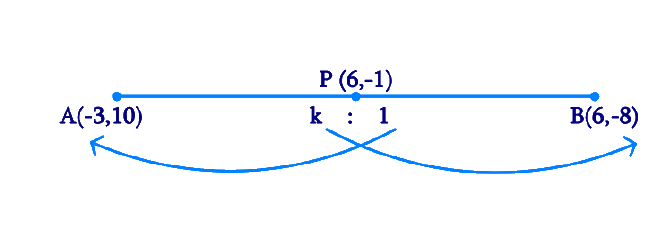# Ex.7.2 Q4 Coordinate Geometry Solution - NCERT Maths Class 10

Go back to  'Ex.7.2'

## Question

Find the ratio in which the line segment joining the points $$(-3, 10)$$ and $$(6, -8)$$ is divided by $$(-1, 6)$$.

Video Solution
Coordinate Geometry
Ex 7.2 | Question 4

## Text Solution

Reasoning:

The coordinates of the point $$P(x, y)$$ which divides the line segment joining the points $$A(x_1, y_1)$$ and $$B(x_2, y_2)$$, internally, in the ratio $$m_1 : m_2$$ is given by the Section Formula.

\begin{align} P(x,y)& \!=\! \left[ {\frac{{mx_2 \! + \! nx_1}}{{m \! + \! n}}\!,\!\frac{{my_2 \! + \! ny_1}}{{m \! + \! n}}} \!\right]\end{align}

What is Known?

The $$x$$ and $$y$$ co-ordinates of the line segment which is divided by the point $$(-1, 6)$$.

What is Unknown?

The ratio in which the line segment joining the points $$(-3, 10)$$ and $$(6, -8)$$ is divided by $$(-1, 6).$$

Steps:

From the figure,Given,

• Let the ratio in which the line segment joining $$A(-3, 10)$$ and $$B(6, -8)$$ is divided by point $$P(-1, 6)$$ be $$k:1$$.

By Section formula

\begin{align} P(x,y)& \!=\! \left[ {\frac{{mx_2 \! + \! nx_1}}{{m \! + \! n}}\!,\!\frac{{my_2 \! + \! ny_1}}{{m \! + \! n}}} \!\right]\;\;\dots(2) \; \end{align}

Therefore,

\begin{align} - 1 &= \frac{{6k - 3}}{{k + 1}}\\ - k - 1 &= 6k - 3\\7k &= 2\end{align}

By Cross Multiplying & Transposing

\begin{align}k &= \frac{2}{7}\end{align}

Hence the point $$P$$ divides $$AB$$ in the ratio $$2:7$$

Learn from the best math teachers and top your exams

• Live one on one classroom and doubt clearing
• Practice worksheets in and after class for conceptual clarity
• Personalized curriculum to keep up with school